# AP Microeconomics : Competition

## Example Questions

← Previous 1 3 4 5 6 7 8 9 10

### Example Question #1 : Perfectly Competitive Labor Markets

The following question will refer to this table: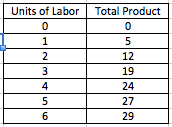What is the marginal product of the fourth unit of labor?

12

19

24

5

5

Explanation:

The marginal product of labor is the change in output that results from an additional unit of labor. In this case, the marginal product of the fourth unit of labor would be the difference between the total output of production with four units of labor and the total output of production with three units of labor, which is 5 units.

### Example Question #1 : Perfectly Competitive Labor Markets

A minimum wage for coal miners will likely result in:

Lower wages for coal miners

A decrease in the supply of coal miners

A shortage of coal miners

An increase in the demand for coal miners

A surplus of coal miners

A surplus of coal miners

Explanation:

Imposing a minimum wage usually causes a price floor in the market. Thus, the labor market for coal miners will likely see a surplus, which typically occurs when there is a price floor. The price will be higher than the equilibrium, which means that the labor supply will be greater than demand.

### Example Question #1 : Perfectly Competitive Markets

The following question is based on this table: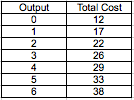What is the total variable cost of producing five goods?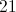Cannot be determined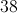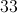Explanation:

Variable costs associated with production are costs that change in proportion to the level of production. By contrast, fixed costs are costs that are not dependent on production level. Fixed costs and variable costs make up a good's total cost of production.

In this case, the total cost of producing zero units is 12. The total variable cost of producing zero units is 0, which means that 12 must be the fixed cost associated with producing this good.

The total cost of producing 5 units is 33. Since total costs subtracted by fixed costs equals its variable costs, the variable cost of producing 5 units must be 21.

### Example Question #3 : Perfectly Competitive Markets

If the total product of labor increases at an increasing rate, _________ .

marginal product of labor is at its maximum.

marginal product of labor is decreasing.

marginal product of labor is increasing.

marginal product of labor is at its minimum.

marginal product of labor is constant.

marginal product of labor is increasing.

Explanation:

Marginal product of labor describes how the total product of labor changes when more labor is hired. If the total product of labor is rising as labor increases, then the marginal product of labor must be increasing.

### Example Question #1 : Competition

A perfectly competitive firm produces at its profit maximizing level. At this point, the marginal product of labor is 7 units per hour and wages are $14 per hour. Which of the following is true? Possible Answers: The cost of producing the good is$2 per unit.

None of the other answers are correct.

The market price of the good is $2 per unit. The market price of the good is$3 per unit.

The cost of producing the good is $3 per unit. Correct answer: The market price of the good is$2 per unit.

Explanation:

To maximize profits, a perfectly competitive firm will hire until its marginal revenue of labor equals its marginal cost of labor. The marginal cost of labor equals the wage rate, which is $14 per hour. The marginal revenue of labor is the marginal product of labor multiplied by the price of the good. Since the marginal product at the profit maximizing level is 7 units per hour, the market price of the good must be$2 (7 units multiplied by $2 equals$14).

### Example Question #5 : Perfectly Competitive Markets

A food safety issue causes a temporary halt in the production of hot dogs at a significant number of firms. What is the expected effect on the equilibrium price and quantity for hot dog buns, assuming a perfectly competitive marketplace?

Price decreases, Quantity decreases

Price increases, Quantity increases

No change

Price increases, Quantity decreases

Price decreases, Quantity increases

Price decreases, Quantity decreases

Explanation:

Hot dogs and hot dog buns are a model pair of complementary goods. Few people buy one without buying the other. You can graph the situation described using supply and demand curves for each market.

In the hot dog market, a significant decrease in supply will result in an inward shift of the supply curve which results in a higher price and fewer hot dogs being bought and sold.

The resulting effect in the market for hot dog buns, with fewer hot dogs being sold, is an inward shift in the demand curve, which results in a decrease in both the market price and quantity sold of hot dog buns.

### Example Question #1 : Competition

If there is a bumper crop in cotton the same year that it becomes very fashionable and consumers begin to demand it over other fabrics, what is the likely effect on the market price and quantity traded?

Price increases, Quantity increases

No change in Price, Quantity increases

Price decreases, Quantity increases

Price indeterminate, Quantity increases

Price increases, no change in Quantity

Price indeterminate, Quantity increases

Explanation:

Graph supply and demand curves to represent the market for cotton. The changes described would result in an outward shift of both the supply and demand curves. The new equilibrium would clearly have an increase in the quantity traded, but the equilibrium price would depend on the slope of the curves. Therefore, without more information, the effect on the market price is indeterminate.

### Example Question #1 : Perfectly Competitive Output Markets

Sugar can be refined from either sugar cane or sugar beets. Which of the following would result in an increase in the market price of sugar cane, assuming perfect competition?

A bumper crop of sugar cane

A bumper crop of sugar beet

Consumers start eating less refined sugar

A sugar beet crop failure

A sugar beet crop failure

Explanation:

A sugar beet crop failure would result in an inward shift of the supply curve in the market for sugar beets, resulting in a higher equilibrium price.

Since sugar beets and sugar cane are substitutes, this would result in greater demand for sugar cane, which would result in a higher price for sugar cane as well.

### Example Question #8 : Perfectly Competitive Markets

Sugar can be refined from either sugar cane or sugar beets. If there is a larger-than-expected harvest in BOTH sugar cane and sugar beets, what is the effect on the equilibrium price and quantity in the market for sugar cane?

Price increases, Quantity increases

Price indeterminate, Quantity decreases

Neither Price nor Quantity can be determined from the information given.

Price indeterminate, Quantity increases

Price decreases, Quantity indeterminate

Price decreases, Quantity indeterminate

Explanation:

First, an increase in the supply of sugar beet results in a lower market price, and therefore lower demand for sugar cane, since they are substitutes. This translates into a lower market price and lower quantity traded for sugar cane.

Second, the increased supply of sugar cane would result in a lower market price and higher quantity traded in sugar cane. Therefore, the market price would definitely decrease, but the direction of the change in quantity cannot be determined.

### Example Question #1 : Perfectly Competitive Markets

Compared to a perfectly competitive market, a monopolist produces...

more of a good and charges a lower price.

more of a good and charges a higher price.

less of a good and charges a lower price.

Unable to determine from the information given.

less of a good and charges a higher price.

less of a good and charges a higher price.

Explanation:

As seen in the graph below, the monopolist faces a downward sloping marginal revenue curve that is steeper than the demand curve. The monopolist produces where MC = MR which results in a higher price and lower output compared to where the marginal cost curve meets the demand curve, which is where equilibrium would be in a perfectly competitive market.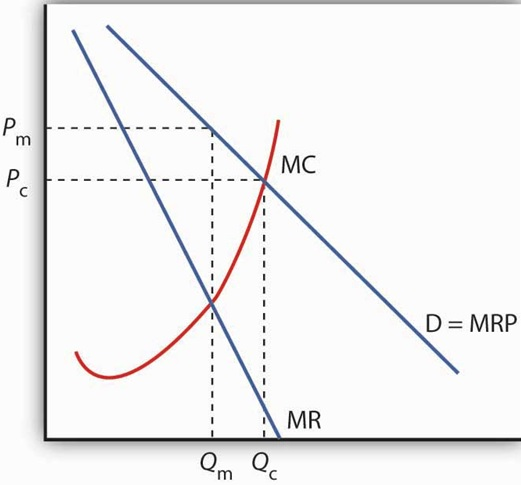← Previous 1 3 4 5 6 7 8 9 10

### All AP Microeconomics Resources